Prof. Bryan Caplan

http://www.gmu.edu/departments/economics/bcaplan

Econ 345

Fall, 1998

Weeks 1-2: Brief Review of Basic Statistics

1. What is Econometrics?
1. Econometrics is the application of statistics to economics.
2. Econometrics uses computers to apply statistics to economic questions.
3. Contrast with economic history.
4. Qualified skepticism about the usefulness of econometrics.
2. Probability
1. Where x is any event,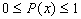. The probability of an event ranges between impossible and certain.
2. Where X is the set of all possible events x,. The probability that some possible event or other occurs is certain.
3. Graphing discrete probability densities; graphing continuous probability densities.
4. Independence: X and Y are independent iff P(X,Y)=P(X)P(Y).
5. Conditional probability: P(X|Y)=P(X,Y)/P(Y).
3. Expected Values
1. E(X) is just the mean or "average" of a random variable X. Formally,.
2. Note:unless X is a constant.
4. Variance and Standard Deviation
1. Var(X)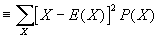. SD(X) is equal to the square root of Var(X). Intuitively, both measure the "spread" of a distribution. If X is a constant, then both SD(X) and Var(X)=0.
2. In practice, Var(X) is a pain to calculate using the above definition. Fortunately, there is extremely useful formula that permits ready calculation: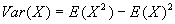.
3. Summing N independent draws from a random variable X has a very interesting property: while the expectation of the average of N draws is simply E(X), the SD(average of N independent draws of X)=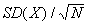. Intuitively, this means that the more independent draws, the more accurate the estimate of E(X) becomes.
5. Covariance and Correlation
1. Both covariance and correlation measure the linear association of two variables: if covariance and correlation for two variables is positive, the two variables are positively associated; if negative, then the two variables are negatively associated. If random variables are independent, then their covariance and correlation is zero.
2. Cov(X,Y); slightly simpler formula: Cov(X,Y)=E(XY)-E(X)E(Y). Covariance ranges over the real numbers.
3. Corr(X,Y)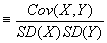. The correlation coefficient ranges between -1 and +1; this makes it much easier to interpret than covariance. If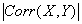is high, then it is possible to make good predictions about one variable if you know the other.
6. Estimating Population Mean and Population Variance
1. If you observe all members of a population, then it is straightforward to calculate the mean and the variance. However, in many cases we observe only PART of the population - and then use what we have seen to estimate what the whole population is like.
2. An easy case: estimating the population mean by simply taking the sample mean.
3. Tougher case: estimating the population variance using:.
4. Why do you divide by (n-1) instead of n? Think about the variance of a single point.
5. Notice that we could have just used ONE observation instead of the sample mean. But that is a bad idea because using more data gives us a lower variance for our estimate. Intuition: remember that the Var(average of N independent draws of X)=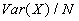.
7. Standard errors, Confidence Intervals, and Hypothesis Testing
1. Terminological note: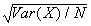is often called the "standard error" of an estimate.
2. Important fact: a sample average of observations from a population less its true mean divided by its standard error has a t-distribution with (n-1) degrees of freedom. In math,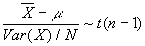.
3. The t-distribution looks very similar to the more familiar Normal distribution, but you need to use it when Var(X) is estimated rather than known. When n is large, the t-distribution becomes approximately Normal.
4. You can use the above formula to construct a Confidence Interval, or range within which the true value of something lies with a certain probability. For example, suppose that we observe 61 dogs' weights, and find that the sample mean is 40 pounds and the sample variance is 15 pounds. Then to construct a 95% Confidence Interval:
1. Plug in the numbers. The sample mean is 40. The sample variance is 15 pounds, so with 61 observations, the standard error is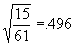. 61-1=60, so we must use the t(60) distribution.
2. Now, go to the t-distribution table. The table shows the values for the right tail, so the extreme left and right tails combined have double the value of the right tail alone.
3. This means that for a 95% C.I., we want the .025 (2.5%) column. For the t(60) distribution, go to the row marked 60.
4. Get the value at the given row and column. It is 2.000.
5. Multiply this number by the standard error - in this case, .496, to get .992.
6. The 95% C.I. here is therefore 40±.992.
5. Hypothesis testing is trivial once you understand C.I.'s.
1. Just plug your hypothesis into the C.I. instead of the sample mean, and see if your observed sample mean lies within the C.I.
2. If your sample mean lies outside the C.I., you "reject the hypothesis." Otherwise you can accept it (or as some prefer to say, "fail to reject it").